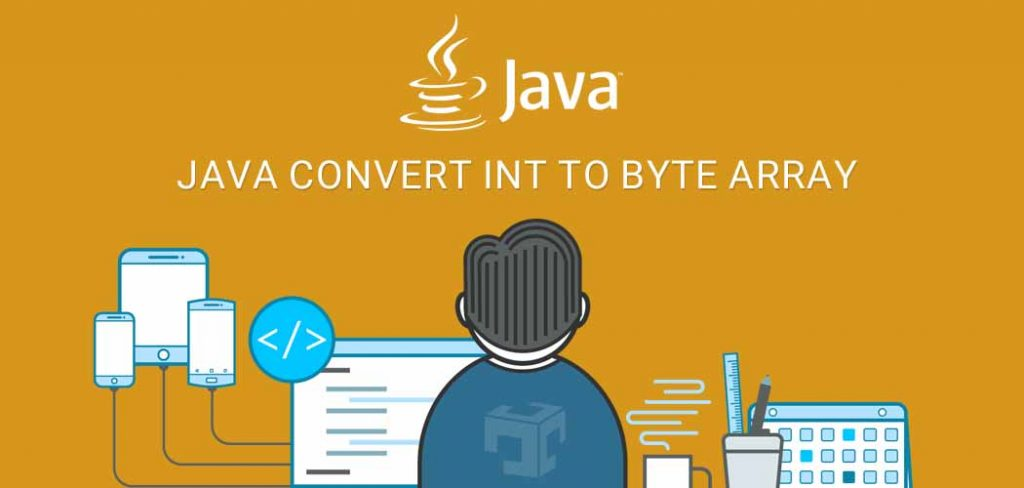# Java Convert int to byte array

## 1. Overview

In this article, we will discuss various techniques of converting int to a byte array and vice versa, int array to byte array and so on.In java int data type take 4 bytes (32 bits) and it’s range is -2,147,483,648 to 2,147,483, 647.

## 2. int to byte array

Byte arrays are commonly used in applications that stream data byte-wise, such as socket connections that send data in byte arrays through TCP or UDP protocols.
It also used in applications that read/write binary files. Here we have discussed four different ways to convert int to byte array in java as below:

### 2.1 BigInteger

BigInteger provides support of all Java’s primitive integer operators and all methods from java.lang.Math.
BigInteger also provides operations for a prime generation, GCD calculation, modular arithmetic, primality testing, bit manipulation etc.. `toByteArray`method of `BigInteger`class is used to convert bigint to byte array.

First, we need to convert int to `BigInteger` using `valueOf` method of BigInterger and then use toByteArray method.

```private byte[] bigIntToByteArray( final int i ) {
BigInteger bigInt = BigInteger.valueOf(i);
return bigInt.toByteArray();
}```

### 2.2 DataOutputStream

Second way to convert int to byte array is using `ByteArrayOutputStream` and `DataOutputStream`.  ByteArrayOutputStream class provide one method `toByteArray` which return underlying written primitive types into a byte array format. Here we have write int to underlying stream and then use the `toByteArray` method to get equivalent byte array.

```private byte[] intToByteArray ( final int i ) throws IOException {
ByteArrayOutputStream bos = new ByteArrayOutputStream();
DataOutputStream dos = new DataOutputStream(bos);
dos.writeInt(i);
dos.flush();
return bos.toByteArray();
}```

### 2.3 ByteBuffer

java.nio provide a facility which help us to work with buffers very efficiently. There are several buffer classes like ByteBuffer, IntBuffer, LongBuffer, MappedByteBuffer etc. under `java.nio` package. Here we have used ByteBuffer class to convert int to byte array using it’s `array` method.

```private byte[] intToBytes( final int i ) {
ByteBuffer bb = ByteBuffer.allocate(4);
bb.putInt(i);
return bb.array();
}```

### 2.4 Shift operations

We can convert int to byte array with the help of shift>> and & operators.

```private static byte[] intToBytes(final int data) {
return new byte[] {
(byte)((data >> 24) & 0xff),
(byte)((data >> 16) & 0xff),
(byte)((data >> 8) & 0xff),
(byte)((data >> 0) & 0xff),
};
}```

## 3. byte array to int

We can convert any byte array to int using below two techniques.

### 3.1 ByteBuffer

Java provides a `ByteBuffer` class to do the same.to convert any byte array, first we need to wrap up using ByteBuffer’s static method wrap and then by calling getInt() method we can get integer value of that byte array.

```private int convertByteArrayToInt(byte[] intBytes){
ByteBuffer byteBuffer = ByteBuffer.wrap(intBytes);
return byteBuffer.getInt();
}```

### 3.2 Shift operations

A second way to convert byte array to int is by using left shift operator and & operator. Left shift operator shifts the number to the left and fills remaining bits with zeros. It is similar to multiplying the number with the power of two.

```private int convertByteArrayToInt(byte[] data) {
if (data == null || data.length != 4) return 0x0;
// ----------
return (int)( // NOTE: type cast not necessary for int
(0xff & data) << 24  |
(0xff & data) << 16  |
(0xff & data) << 8   |
(0xff & data) << 0
);
}```

## 4. int array to byte array

Now we can convert any int array to byte array using our earlier learning of int to byte array. In below example, we have iterate of all the elements from array, convert it to a byte array and prepare final byte array.

```private byte[] convertIntArrayToByteArray(int[] data) {
if (data == null) return null;
// ----------
byte[] byts = new byte[data.length * 4];
for (int i = 0; i < data.length; i++)
System.arraycopy(convertIntToByteArray(data[i]), 0, byts, i * 4, 4);
return byts;
}```

## 5. byte array to int array

Similarly when we converting byte array to int array, first we need to calculate the length of an array. Prepare four bytes chunk and convert it to integer and store it in an array.

```public int[] convertByteArrayToIntArray(byte[] data) {
if (data == null || data.length % 4 != 0) return null;
// ----------
int[] ints = new int[data.length / 4];
for (int i = 0; i < ints.length; i++)
ints[i] = ( convertByteArrayToInt(new byte[] {
data[(i*4)],
data[(i*4)+1],
data[(i*4)+2],
data[(i*4)+3],
} ));
return ints;
}```

## 5. Conclusion

In this article we have discussed various techniques of converting byte array to int, int to byte array, int array to byte array and byte array to int array using ByteBuffer,DataInputStream,DataOutputStream and some custom logic.

Refer ByteBuffer , DataInputStream for more details.

Java is a broadly used programming language, that enables developers to create web applications. byte arrays are a type of data that Java can handle easily. When you want to convert an int value to a byte array, you can use the static method ByteArray.toByteArray(). This method takes an int input and returns a byte array representation of the number.